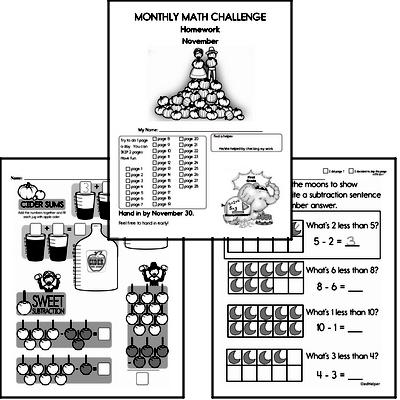Mental Math Workbooks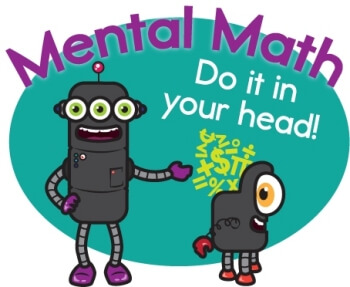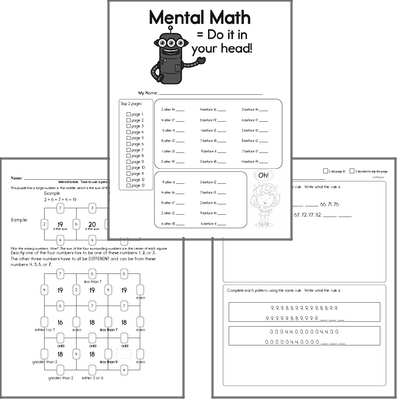Mental Math Workbooks

First Grade Math Worksheets Activity Workbook - No Prep and Not Boring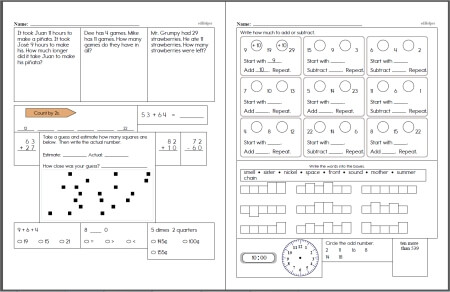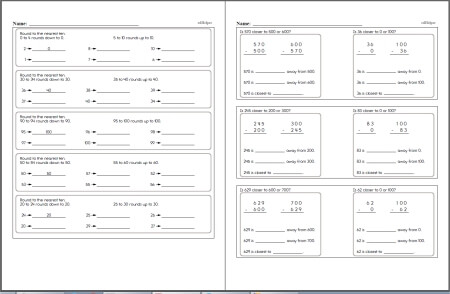First Grade Math Book New First Grade Math Workbook Created Each book contains word problems, mixed math, and other first grade review materials. Use it each week for daily practice in your classroom.

Daily First Grade Math and Critical Thinking Practice

First Grade Daily Homework and Practice Pages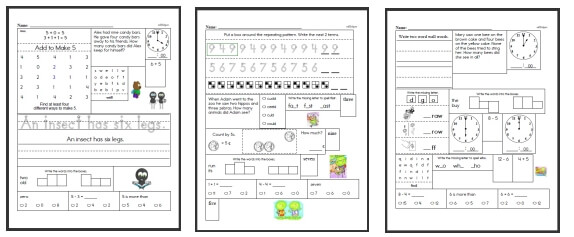First Grade Daily Homework

Level 1: Easiest (First 2 months of school)
Level 2: Next 3 months of school
Level 3: Next 3 months of school
Level 4: Entire Grade (Final months of school)

Weekly Word Problems
Last week's first grade math word problems
This week's first grade math word problems
Next week's first grade math word problems
Daily Review: Customize math review skills with word problems and language arts

Kindergarten and Preschool Math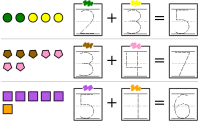Preschool to Kindergarten Math

Counting

Number Lines

Comparing Numbers

Skip Counting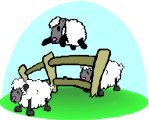Skip Counting Reading Comprehensions (grades 1-2 reading level)

Hundreds Chart

Hundreds Chart Pieces Puzzle

Counting (black and white pictures)
Numbers: 0-9
Circle the set that has fewer
Circle the set that has more
Numbers 1-5: Count and write the number (gives answer to trace over)
Numbers 1-5: Count and write the number
Numbers 6-9: Count and write the number (gives answer to trace over)
Numbers 6-9: Count and write the number
Counting Mixed Review

Numbers: 11-19
Numbers 11-15: Count and write the number (gives answer to trace over)
Numbers 11-15: Count and write the number
Numbers 16-19: Count and write the number (gives answer to trace over)
Numbers 16-19: Count and write the number
Counting Mixed Review

Counting (color)
Numbers: 0-9
Circle the set that has fewer
Circle the set that has more
Numbers 1-5: Count and write the number (gives answer to trace over)
Numbers 1-5: Count and write the number
Numbers 6-9: Count and write the number (gives answer to trace over)
Numbers 6-9: Count and write the number
Counting Mixed Review

Numbers: 11-19
Numbers 11-15: Count and write the number (gives answer to trace over)
Numbers 11-15: Count and write the number
Numbers 16-19: Count and write the number (gives answer to trace over)
Numbers 16-19: Count and write the number
Counting Mixed Review

Ordering Numbers
Numbers: 0-10
Fill in the missing numbers (Just before, after, and between)
Greater than, less than, and equal to (circle the words)
Circle the larger number
Circle the smaller number
Order 3 numbers (from smallest to largest)
Order 3 numbers (from largest to smallest)
Order 4 numbers
Ordering Mixed Review

Numbers: 11-20
Fill in the missing numbers (Just before, after, and between)
Greater than, less than, and equal to (circle the words)
Circle the larger number
Circle the smaller number
Order 3 numbers (from smallest to largest)
Order 3 numbers (from largest to smallest)
Order 4 numbers
Ordering Mixed Review

Numbers: 0 to 20
Fill in the missing numbers (Just before, after, and between)
Greater than, less than, and equal to (circle the words)
Circle the larger number
Circle the smaller number
Order 3 numbers (from smallest to largest)
Order 3 numbers (from largest to smallest)
Order 4 numbers
Ordering Mixed Review

Numbers: 0 to 50
Fill in the missing numbers (Just before, after, and between)
Greater than, less than, and equal to (circle the words)
Circle the larger number
Circle the smaller number
Order 3 numbers (from smallest to largest)
Order 3 numbers (from largest to smallest)
Order 4 numbers
Ordering Mixed Review

Numbers: 0 to 99
Fill in the missing numbers (Just before, after, and between)
Greater than, less than, and equal to (circle the words)
Circle the larger number
Circle the smaller number
Order 3 numbers (from smallest to largest)
Order 3 numbers (from largest to smallest)
Order 4 numbers
Ordering Mixed Review

Ordering and Counting
 Mazes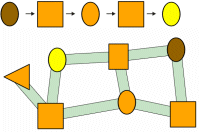Number Mazes and Shapes Mazes

 Matching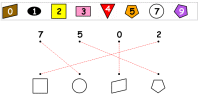Matching Shapes, Numbers, and Colors

 Combined Shapes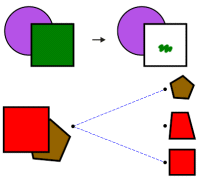Combined Shapes: Coloring and Matching

 Ordering Animals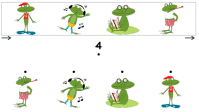Ordering Animals

 Ordering Shapes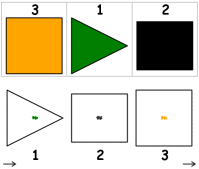Ordering Shapes
 Grids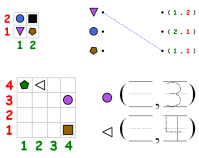Grids: Shapes, Positions, Writing Numbers

 Greater and Less Than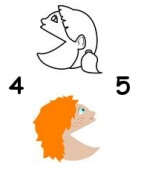Greater and Less Than

Odd and Even Numbers

Subtraction and Introduction to Subtraction

Blocks (black and white)
Find sum (2-7 blocks)
Fill in the missing number (2-7 blocks)
Complete the addition fact (2-7 blocks)
Find sum (6-9 blocks)
Fill in the missing number (6-9 blocks)
Complete the addition fact (6-9 blocks)

Blocks (color)
Find sum (2-7 blocks)
Fill in the missing number (2-7 blocks)
Complete the addition fact (2-7 blocks)
Find sum (6-9 blocks)
Fill in the missing number (6-9 blocks)
Complete the addition fact (6-9 blocks)

Dominoes
Fill in the blanks (sum of 2-9)
Complete the addition fact (sum of 2-9)
Fill in the blanks (sum of 6-12)
Complete the addition fact (sum of 6-12)

Fill in the missing numbers

Word search: adding the number 1
Word search: adding the number 2
Word search: adding the number 3

Word problems and review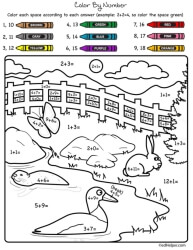Color by Number Addition

CountingCounting (part 1)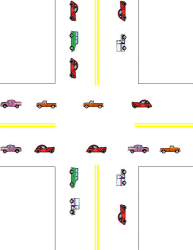Counting (part 2)

Math Facts

Addition Math Facts: Numbers 0 to 9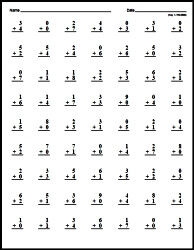Addition Facts: Numbers 0 to 9 Cross out the incorrect facts Make Custom Quick Math Facts Printable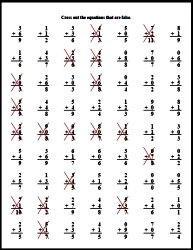Addition Facts: Focusing on a number

Subtraction: Numbers 0 to 10
Subtracting the number 1
Subtracting the number 2
Subtracting the number 3
Subtracting the number 4
Subtracting the number 5
Subtracting the number 6
Subtracting 7-10
Subtracting 1-3
Subtracting 2-4
Subtracting 2-5
Subtracting 1-9

Fill in the missing numbers
Subtracting 1-3
Subtracting 2-4
Subtracting 2-5
Subtracting 4-7
Subtracting 3-8
Subtracting 1-9

Find subtraction facts
Word search: subtract the number 1
Word search: subtract the number 2
Word search: subtract the number 3
Word search: subtract the number 4
Word search: subtract the number 5
Word Search: subtracting 1-3
Word Search: subtracting 3-5
Word Search: subtracting 4-9

Word problems and review
Subtraction word problems
Subtraction (Numbers 0-10) Mixed Review

Subtraction Math Facts: Numbers 0 to 9Subtraction Facts: Numbers 0 to 9 Cross out the incorrect facts Make Custom Quick Math Facts PrintableSubtraction Facts: Focusing on a number
Subtraction with the number 1
Subtraction with the number 2
Subtraction with the number 3
Subtraction with the number 4
Subtraction with the number 5
Subtraction with the number 6
Subtraction with the number 7
Subtraction with the number 8
Subtraction with the number 9

Patterns - Black and White
Select the shape that comes next (rectangle, square, circle, and triangle)
2 different shapes used per problem
Patterns mix different colored shapes
8-12 shapes per pattern
3 different shapes used
2-3 shapes used with different colored shapes
Includes shapes with patterns (11-14 shapes)
13-17 shapes per pattern

Draw the shapes to complete each pattern
8-12 shapes, fill in the blanks at the end
8-12 shapes, fill in the blanks in the middle
8-12 shapes, fill in the blanks at the start
9-13 shapes, one missing space (randomly placed)
9-16 shapes, two consecutive blanks (randomly placed)
9-16 shapes, two missing spaces (randomly placed)
Patterns Mixed Review

Patterns using basic shapes and also additional shapes with 5-8 sides
Select the shape that comes next - sheet #1
Select the shape that comes next - sheet #2
Select the shape that comes next - sheet #3
Select the shape that comes next - sheet #4
Select the shape that comes next - sheet #5
Patterns Mixed Review

Patterns - Color
Select the shape that comes next (rectangle, square, circle, and triangle)
2 different shapes used per problem
Patterns mix different colored shapes
8-12 shapes per pattern
3 different shapes used
2-3 shapes used with different colored shapes
Includes shapes with patterns (11-14 shapes)
13-17 shapes per pattern

Draw the shapes to complete each pattern
8-12 shapes, fill in the blanks at the end
8-12 shapes, fill in the blanks in the middle
8-12 shapes, fill in the blanks at the start
9-13 shapes, one missing space (randomly placed)
9-16 shapes, two consecutive blanks (randomly placed)
9-16 shapes, two missing spaces (randomly placed)
Patterns Mixed Review

Patterns using basic shapes and also additional shapes with 5-8 sides
Select the shape that comes next - sheet #1
Select the shape that comes next - sheet #2
Select the shape that comes next - sheet #3
Select the shape that comes next - sheet #4
Select the shape that comes next - sheet #5
Patterns Mixed Review

Addition with Blocks (includes the total block count)
Find the sum
Fill in one number

Find the sum
Fill in one number

Doubles and Doubles + 1
Mixed vertical and horizontal
Fill in the missing numbers
Word search

Blocks: find the sum
Blocks: fill in one number
Mixed vertical and horizontal

Addition with sums from 11 through 15
Fill in the missing numbers
Word search
Adding three numbers: vertical and horizontal
Adding three numbers: fill in the missing numbers
Word problems

Addition with sums from 11 through 19
Fill in the missing numbers
Word search
Adding three numbers: vertical and horizontal
Adding three numbers: fill in the missing numbers
Word problems

Review

Subtraction Through 20
Subtraction with Blocks: subtracting 1-5 (includes the answer)
Find the difference
Fill in one number
Complete the subtraction fact

Subtraction with Blocks: subtracting 1-5
Find the difference
Fill in one number
Complete the subtraction fact

Subtraction with Blocks: subtracting 6-10 (includes the answer)
Find the difference
Fill in one number
Complete the subtraction fact

Subtraction with Blocks: subtracting 6-10
Find the difference
Fill in one number
Complete the subtraction fact

Subtracting 1 to 4 from 10 to 19
Subtracting 5 to 9 from 10 to 19
1 addition and 2 subtraction facts
1 subtraction and 2 addition facts
Mixed

Subtracting from 16 through 20
Horizontal and vertical subtraction
Vertical subtraction
Horizontal subtraction
Fill in the missing numbers
Word search
Word problems

Fill in the missing numbers (number is same for each set)
Fill in the missing numbers

Subtracting from 11 through 20
Horizontal and vertical subtraction
Vertical subtraction
Horizontal subtraction
Fill in the missing numbers
Word search
Word problems

Review
Subtraction Through 20 Mixed Review

Addition and Subtraction Through 20 Review
Blocks
Find the difference
Fill in one number
Complete the subtraction fact

Mixed
Horizontal and vertical addition and subtraction
Fill in the missing numbers
Word search

Word Problems and Review
Addition and subtraction through 20 word problems
Addition and Subtraction Through 20 Mixed Review

Symmetry and Lines of Symmetry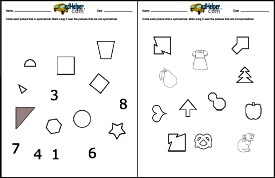Symmetry and Lines of Symmetry

Slides, Turns, and Flips

Fractions
One half - circle the shape
One half - color
One third - circle the shape
One third - color
One fourth - circle the shape
One fourth - color
What fraction does the colored part show? Circle the fraction
Color one part
Draw lines to show the fraction
Parts of a group
More parts of a group
Symmetry
Fractions and pizza

Fractions Review
Fractions Review
Fractions Review (larger review and includes pizza word problems)

Place Value

Place Value to 99: Ones and Tens
Numbers 11 to 49
Graphics: count and write the number of ones and tens
Given a number: write the number of ones and tens
Given ones and tens: write the number
Fill in the missing number
Circle the larger number
Circle the smaller number

Numbers 51 to 99
Graphics: count and write the number of ones and tens
Given a number: write the number of ones and tens
Given ones and tens: write the number
Fill in the missing number
Circle the larger number
Circle the smaller number

Numbers 1 to 99
Graphics: count and write the number of ones and tens
Given a number: write the number of ones and tens
Given ones and tens: write the number
Fill in the missing number
Circle the larger number
Circle the smaller number

Review
Place Value to 99: Ones and Tens Review

Money (black and white coins)
Counting (each problem only uses one type of coin)
Counting pennies
Counting nickels
Counting dimes
Mixed counting
Mixed counting multiple choice
Total value of coins

Nickels and Pennies
Counting
Multiple choice
Write total value (3-9 coins)
Write total value (7-12 coins)
Circle the coins that match the price
Draw a certain number of cents using a given number of coins
Fill in the missing numbers

Dimes and Pennies
Counting
Multiple choice
Write total value (3-9 coins)
Write total value (7-12 coins)
Circle the coins that match the price
Draw a certain number of cents using a given number of coins
Fill in the missing numbers

Pennies, Nickels, and Dimes
Counting
Multiple choice
Write total value (3-9 coins)
Write total value (7-12 coins)
Circle the coins that match the price
Draw a certain number of cents using a given number of coins
Fill in the missing numbers

Review
Money Review: Pennies, Nickels, and Dimes

Pennies, Nickels, and Quarters
Counting
Multiple choice
Write total value (3-9 coins)
Write total value (7-12 coins)
Circle the coins that match the price
Draw a certain number of cents using a given number of coins
Fill in the missing numbers

Pennies, Nickels, Dimes, and Quarters
Counting
Multiple choice
Write total value (3-9 coins)
Write total value (7-12 coins)
Circle the coins that match the price
Draw a certain number of cents using a given number of coins
Fill in the missing numbers

Review
Money Review: Pennies, Nickels, Dimes, and Quarters

Length and Weight
Length (black and white graphics)
Circle the object with the longest side
Number the objects in order from shortest to longest
Number the objects in order from longest to shortest
Estimate (inches and feet)
Estimate (centimeters and meters)
Weight (black and white graphics)
Circle the object that is heavier
Circle the object that is lighter
Number the objects in order from lightest to heaviest
Number the objects in order from heaviest to lightest
Estimate (ounces and pounds)
Estimate (grams and kilograms)
Review (black and white graphics)
Length and weight review (black and white graphics)

Length (color graphics)
Circle the object with the longest side
Number the objects in order from shortest to longest
Number the objects in order from longest to shortest
Estimate (inches and feet)
Estimate (centimeters and meters)
Weight (color graphics)
Circle the object that is heavier
Circle the object that is lighter
Number the objects in order from lightest to heaviest
Number the objects in order from heaviest to lightest
Estimate (ounces and pounds)
Estimate (grams and kilograms)
Review (color graphics)
Length and weight review (color graphics)

Capacity
Order Capacity: Order the objects from smallest capacity to greatest capacity
Order Capacity: Order the objects from greatest capacity to smallest capacity
Circle the container that holds more
Circle the container that holds less

Cups and Pints
Circle the set which holds more
Circle the set which holds less
Which holds more? (more difficult)
Which holds less? (more difficult)

Pints and Quarts
Circle the set which holds more
Circle the set which holds less
Which holds more? (more difficult)
Which holds less? (more difficult)

Cups and Quarts
Circle the set which holds more
Circle the set which holds less
Which holds more? (more difficult)
Which holds less? (more difficult)

Cups, Pints, and Quarts
Circle the set which holds more
Circle the set which holds less
Which holds more? (more difficult)
Which holds less? (more difficult)

Quarts and Gallons
Circle the set which holds more
Circle the set which holds less
Which holds more? (more difficult)
Which holds less? (more difficult)

Pints, Quarts, and Gallons
Circle the set which holds more
Circle the set which holds less
Which holds more? (more difficult)
Which holds less? (more difficult)

Mix
Cups and pints
Cups, pints, and quarts
Cups, pints, quarts, and gallons

Temperature
Is the temperature hot or cold in the picture?
Color the thermometer to show the temperature
Color the thermometer to show the temperature

Capacity Review
Capacity mixed review

Adding a one-digit numbers to two-digit numbers (No Regrouping)
Adding tens and ones with blocks
Vertical addition - 2 digits on top and 1 digit on bottom
Vertical addition - 1 digit on top and 2 digits on bottom
Vertical addition - 2 digits and 1 digit (mixed)
Fill in the missing number in each problem
Fill in the missing numbers

Adding tens and ones with blocks
Fill in the missing number in each problem
Fill in the missing numbers

Word problems and review (No Regrouping)
Two-Digit addition Mixed Review (No Regrouping)

Subtracting Two-Digit Numbers (No Regrouping)
Subtracting a one-digit number from a two-digit number (No Regrouping)
Subtracting tens and ones with blocks
Vertical subtraction - 2 digits on top and 1 digit on bottom
Fill in the missing number in each problem
Subtracting cents
Horizontal and vertical subtraction
Vertical subtraction
Horizontal subtraction
Fill in the missing numbers

Two-Digit Subtraction (No Regrouping)
Subtracting tens and ones with blocks
Vertical subtraction
Fill in the missing number in each problem
Subtracting cents
Horizontal and vertical subtraction
Vertical subtraction
Horizontal subtraction
Fill in the missing numbers

Word problems and review (No Regrouping)
Two-Digit subtraction word problems
Two-Digit Subtraction Mixed Review (No Regrouping)

Adding and Subtracting Two-Digit Numbers (No Regrouping)
Adding and Subtracting with one one-digit number and one two-digit number (No Regrouping)
Adding and subtracting tens and ones with blocks
Vertical addition and subtraction - 2 digits on top and 1 digit on bottom
Vertical addition and subtraction - 1 digit on top and 2 digits on bottom
Vertical addition and subtraction - 2 digits and 1 digit (mixed)
Fill in the missing number in each problem
Horizontal and vertical addition and subtraction
Fill in the missing numbers

Two-Digit Addition and Subtraction (No Regrouping)
Adding and subtracting tens and ones with blocks
Fill in the missing number in each problem
Horizontal and vertical addition and subtraction
Fill in the missing numbers

Word problems and review (No Regrouping)
Two-Digit addition and subtraction word problems
Two-Digit addition and subtraction Mixed Review (No Regrouping)

Telling Time
Hour
Write the time (____ o'clock)
Write the time (____:____)
Write the time two ways
Show the time on the clock
Write the time two ways (smaller clocks)

Half-Hour
Write the time (half past ____)
Write the time (____:____)
Write the time two ways
Show the time on the clock
Write the time two ways (smaller clocks)

Mixed: Hour and Half-Hour (larger clocks)
Write the time (half past ____ and ____ o'clock)
Write the time (____:____)
Write the time two ways
Show the time on the clock

Mixed: Hour and Half-Hour (smaller clocks)
Write the time (half past ____ and ____ o'clock)
Write the time (____:____)
Write the time two ways
Show the time on the clock
Circle the correct time (2 choices)

Review
Telling Time Mixed Review